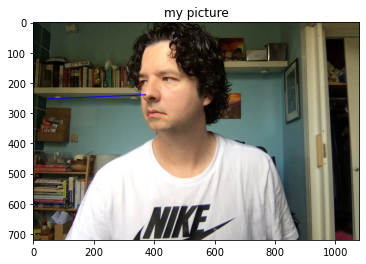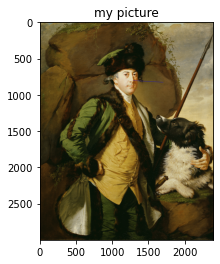Earlier this year I was working on a personal project where I was automating the adding of surgical masks to faces in supplied images (repo here) and an issue I was having was that depending on the direction someone was facing in an image, we’d need to apply the mask image facing the same direction otherwise the effect didn’t look convincing at all.

To help me understand how to apply DLIB’s methodology for head pose detection where I found the documentation not simple, I created this notebook which demonstrates the steps and gets it working in Jupityr.

In :
```import cv2
import numpy as np
import dlib
import imutils
from matplotlib import pyplot as plt

detector = dlib.get_frontal_face_detector()
predictor = dlib.shape_predictor('shape_predictor_68_face_landmarks.dat')

#print(cv2.__version__)
print(dlib.__version__)
print(cv2.__version__)
print(np.__version__)
print(imutils.__version__)
```
```19.19.0
4.2.0
1.18.4
0.5.3
```
In :
```def get_face_stats(im):
size = im.shape
gray  = cv2.cvtColor(im, cv2.COLOR_BGR2GRAY)
rects = detector(gray, 0)
if(len(rects) == 0):
"no face found"
for rect in rects:
face = predictor(gray, rect)
return get_pose_data(size,face)
```
In :
```def get_pose_data(size, face):
#print(type(face))
shape0 = np.array(imutils.face_utils.shape_to_np(face))

image_points = np.array([
(shape0[30, :]),     # Nose tip
(shape0[8,  :]),     # Chin
(shape0[36, :]),     # Left eye left corner
(shape0[45, :]),     # Right eye right corne
(shape0[48, :]),     # Left Mouth corner
(shape0[54, :])      # Right mouth corner
], dtype="double")

# 3D model points.
model_points = np.array([
(0.0, 0.0, 0.0),             # Nose tip
(0.0, -330.0, -65.0),        # Chin
(-225.0, 170.0, -135.0),     # Left eye left corner
(225.0, 170.0, -135.0),      # Right eye right corne
(-150.0, -150.0, -125.0),    # Left Mouth corner
(150.0, -150.0, -125.0)      # Right mouth corner
])

# Camera internals
focal_length = size
center = (size/2, size/2)
camera_matrix = np.array(
[[focal_length, 0, center],
[0, focal_length, center],
[0, 0, 1]], dtype = "double"
)
print ("Camera Matrix :\n {0}".format(camera_matrix))

dist_coeffs = np.zeros((4,1)) # Assuming no lens distortion
(success, rotation_vector, translation_vector) = cv2.solvePnP(model_points, image_points, camera_matrix, dist_coeffs, flags=cv2.SOLVEPNP_UPNP)
print ("Rotation Vector:\n {0}".format(rotation_vector))
print ("Translation Vector:\n {0}".format(translation_vector))
return {"im":im,"rotation_vector":rotation_vector,"translation_vector":translation_vector,"camera_matrix":camera_matrix,"dist_coeffs":dist_coeffs, "image_points":image_points}
```
In :
```def draw_pose_from_stats(stats):
# Project a 3D point (0, 0, 1000.0) onto the image plane.
# We use this to draw a line sticking out of the nose
(nose_end_point2D, jacobian) = cv2.projectPoints(np.array([(0.0, 0.0, 1000.0)]), stats["rotation_vector"], stats["translation_vector"], stats["camera_matrix"], stats["dist_coeffs"])
im = stats["im"]
image_points = stats["image_points"]
for p in image_points:
cv2.circle(im, (int(p), int(p)), 3, (0,0,255), -1)

p1 = ( int(image_points), int(image_points))
p2 = ( int(nose_end_point2D), int(nose_end_point2D))

cv2.line(im, p1, p2, (255,0,0), 2)
img = cv2.cvtColor(im, cv2.COLOR_BGR2RGB)
print(categorize_look(stats))
plt.imshow(img)
plt.title('my picture')
plt.show()
```
In :
```def categorize_look(stats):
lr = stats["rotation_vector"]
print(lr)
ud = stats["rotation_vector"]
lr_boundry = 0.6
ud_boundry = 0.1
lr_cat = "centre"
if(lr > lr_boundry):
lr_cat = "right"
elif(lr < (0- lr_boundry)):
lr_cat = "left"
ud_cat = "level"
if(ud > ud_boundry):
ud_cat = "up"
elif(ud < (0- lr_boundry)):
ud_cat = "down"
return [ud_cat,lr_cat]
```
In :
```def draw_pose(im):
stats = get_face_stats(im)
draw_pose_from_stats(stats)
```
In :
```im = cv2.imread("faces/centre.jpg");
draw_pose(im)
```
```Camera Matrix :
[[1.08e+03 0.00e+00 5.40e+02]
[0.00e+00 1.08e+03 3.60e+02]
[0.00e+00 0.00e+00 1.00e+00]]
Rotation Vector:
[[3.09883941]
[0.08189339]
[0.30102425]]
Translation Vector:
[[-117.3853116 ]
[-323.95459013]
[3180.11352133]]
0.30102424855340343
['level', 'centre']
```In :
```im = cv2.imread("faces/up.jpg");
draw_pose(im)
draw_pose(im)
```
```Camera Matrix :
[[1.08e+03 0.00e+00 5.40e+02]
[0.00e+00 1.08e+03 3.60e+02]
[0.00e+00 0.00e+00 1.00e+00]]
Rotation Vector:
[[2.81478925]
[0.13779494]
[0.01350059]]
Translation Vector:
[[ -81.85361553]
[-610.59855207]
[3543.69749889]]
0.013500593980314217
['up', 'centre']
``````Camera Matrix :
[[1.08e+03 0.00e+00 5.40e+02]
[0.00e+00 1.08e+03 3.60e+02]
[0.00e+00 0.00e+00 1.00e+00]]
Rotation Vector:
[[-2.74984966]
[-0.14984427]
[-0.83368464]]
Translation Vector:
[[ -33.22084057]
[-107.19381794]
[2758.37785505]]
-0.8336846435970481
['level', 'left']
```In :
```im = cv2.imread("faces/half_right.jpg");
draw_pose(im)
```
```Camera Matrix :
[[1.08e+03 0.00e+00 5.40e+02]
[0.00e+00 1.08e+03 3.60e+02]
[0.00e+00 0.00e+00 1.00e+00]]
Rotation Vector:
[[-2.91447087]
[ 0.07673136]
[ 0.97716504]]
Translation Vector:
[[-481.18420972]
[-313.95696613]
[3178.72098441]]
0.977165037863125
['level', 'right']
```In [ ]:
```
```
In :
```im = cv2.imread("faces/half_left.jpg");
draw_pose(im)
```
```Camera Matrix :
[[1.08e+03 0.00e+00 5.40e+02]
[0.00e+00 1.08e+03 3.60e+02]
[0.00e+00 0.00e+00 1.00e+00]]
Rotation Vector:
[[-2.92937943]
[-0.1548573 ]
[-1.02445005]]
Translation Vector:
[[ 199.81396435]
[-320.05507442]
[3123.49724169]]
-1.0244500543051114
['level', 'left']
```In :
```im = cv2.imread("faces/sideface.jpg");
draw_pose(im)
```
```Camera Matrix :
[[2.394e+03 0.000e+00 1.197e+03]
[0.000e+00 2.394e+03 1.500e+03]
[0.000e+00 0.000e+00 1.000e+00]]
Rotation Vector:
[[-2.63781731]
[ 0.13787184]
[-1.10061914]]
Translation Vector:
[[  187.64741668]
[-1427.63309647]
[ 4978.81513772]]
-1.100619135925297
['up', 'left']
```In :
```im = cv2.imread("faces/sideface2.jpg");
draw_pose(im)
```
```Camera Matrix :
[[2.394e+03 0.000e+00 1.197e+03]
[0.000e+00 2.394e+03 1.500e+03]
[0.000e+00 0.000e+00 1.000e+00]]
Rotation Vector:
[[-2.57494732]
[-0.16653315]
[ 1.04713118]]
Translation Vector:
[[ -190.96108571]
[-1427.23587634]
[ 4983.18647987]]
1.0471311771225094
['level', 'right']
```